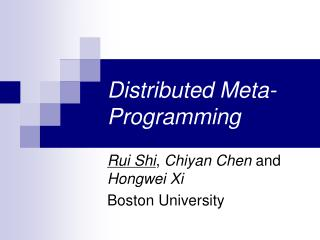Download PresentationDistributed Meta-ProgrammingDistributed Meta-Programming - PowerPoint PPT Presentation

Download PresentationDistributed Meta-Programming
An Image/Link below is provided (as is) to download presentation

Download Policy: Content on the Website is provided to you AS IS for your information and personal use and may not be sold / licensed / shared on other websites without getting consent from its author. While downloading, if for some reason you are not able to download a presentation, the publisher may have deleted the file from their server.

- - - - - - - - - - - - - - - - - - - - - - - - - - - E N D - - - - - - - - - - - - - - - - - - - - - - - - - - -
Presentation Transcript

1. Distributed Meta-Programming Rui Shi, Chiyan Chen and Hongwei Xi Boston University

2. Outline • MP vs. DMP • Typeful Code Representation • The Language dist • The Language +dist • Discussion and Conclusion

3. Meta-Programming (define (run code) (eval code nil)) (define (power n x)(if (= n 0) 1 `(* ,x ,(power (- n 1) x)))) ;;; (power 2 ‘x) yields (* x (* x 1)) (define square(run `(lambda (x) ,(power 2 ‘x)))) ;;; (power 3 ‘y) yields (* y (* y (* y 1))) (define cube(run `(lambda (y) ,(power 3 ‘y))))

4. Distributed Meta-Programming Run-time code generation Distributed execution Code Code

5. Outline • MP vs. DMP • Typeful Code Representation • The Language dist • The Language +dist • Discussion and Conclusion

6. Un-typed Code Representation • No guarantee on the safety of generated code. • Code can be syntactically ill-formed or ill-typed, whichlead to run-time errors when executed at remote sites. • Issues of locality and heterogeneity of resources • Different sites may have different resources and thus likely to provide different services. `(“abc” + 10) (ill-typed) `(( y.x) 10) (open code) f : (Int ! Int)@L `(f 100) L L’

7. Typeful Code Representation (T.C.R) • The types of object programs can be reflected in the types of meta programs • E.g. code of type h, T i represents an object program of type T under the type environment . • The type of the code also reflects where it should be sent for execution. • E.g. code of type h L, , T ican be sent to location Lfor execution. • The compiler can statically guarantee that only well-typed code can be constructed at run-time and sent to proper locations for execution.

8. Outline • MP vs. DMP • Typeful Code Representation • The Language dist • The Language +dist • Discussion and Conclusion

9. The Language dist

10. First-Order Code Constructors For example, the term  x. y. y (x) can be represented as: which can be of type (with static variables properly instantiated) Lam (Lam (App (One) (Shi (One)))) 8 . h, , int ! (int ! int) ! int i

11. Some Primitive Functions

12. Constant Messages • Constant messages refer to the messages which can be interpreted at all sites. • Example: perform addition of two integersat remote site

13. An Example in dist f : (Int ! Int)@L `(f 100) L rgetint (L, rexec (L, App (Lift (n2m f)), Lift 100) )) of type h L, , int i

14. Outline • MP vs. DMP • Typeful Code Representation • The Language dist • The Language +dist • Discussion and Conclusion

15. The Language +dist • We extend dist to +dist with some language constructs adopted from meta-programming (supported in Scheme and MetaML): • `(e) for the code representation of e • ^(e) for spliceing the code e into some context • %(¢) is a shorthand for ^(Lift(¢))

16. An Example in Concrete Syntax of +dist typedef CodeType (L: loc) = <L, nil, (int -> int) -> int> fun rZeroFind2{L:loc} (L:loc(L)) (n: (int -> int) @ L) : int = let val zeroFindCode : CodeType (L) = `(lam f => (fix aux i => if f (i) = 0 then i else aux (i+1)) 0) in rexecInt (L, `(^zeroFindCode %(n2m n))) end

17. Outline • MP vs. DMP • Typeful Code Representation • The Language dist • The Language +dist • Discussion and Conclusion

18. Discussion • We have a transformation scheme from +dist to dist to justify the soundness of +dist . • We have a process to elaborate programs (partial type inference)in the concrete syntax to the formal syntax of +dist • DMP is implemented as a part of ATS (Applied Type System) framework.

19. Conclusion • A simple and general approach to support typed mobile computing. • Combines meta-programming with distributed programming in a coherent manner. • In future, we are planning to study issues such as exception handling and distributed garbage collection in this context.

20. Thank You!